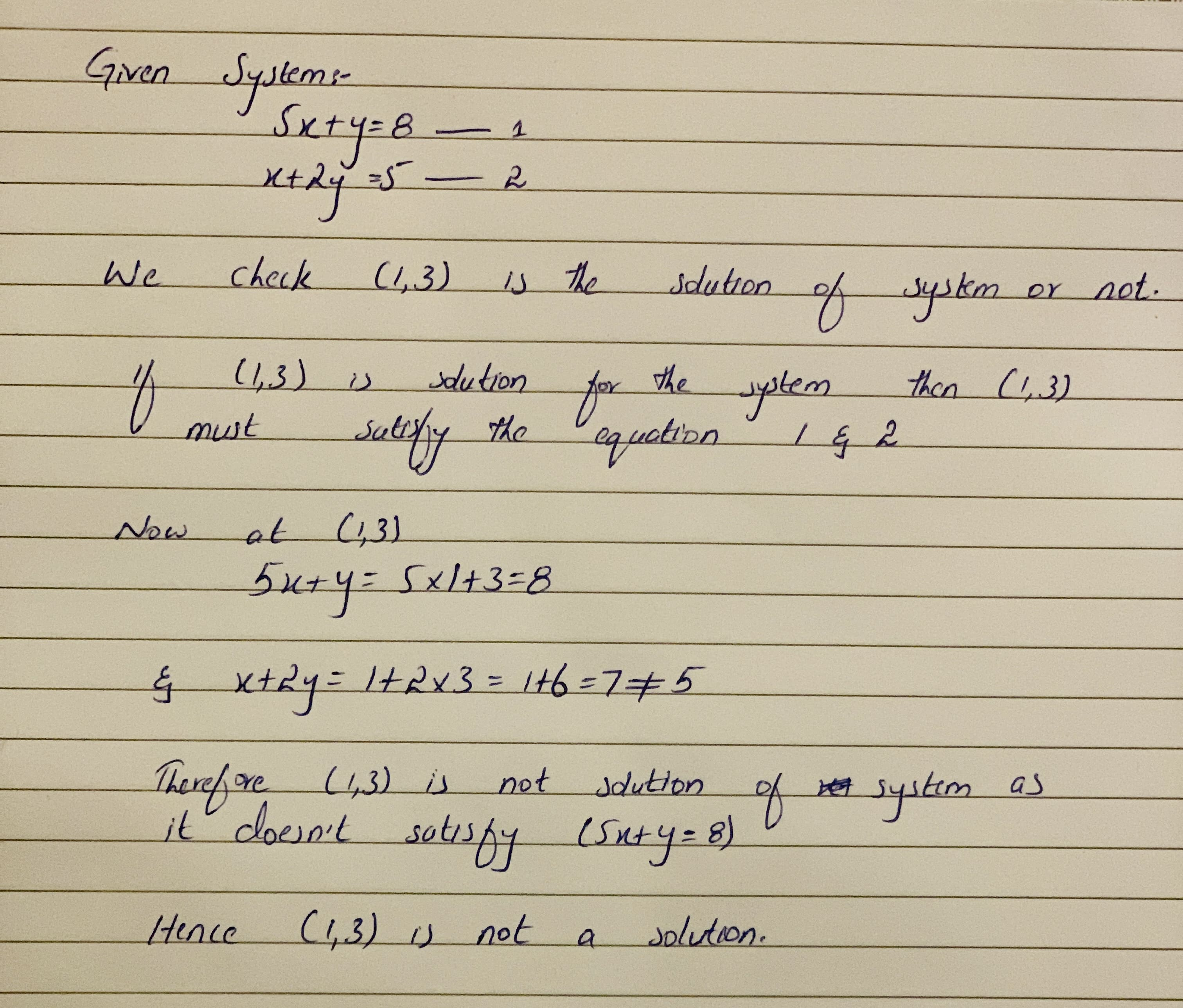# Determine if (1,3) is a solution to the given system of linear equations. 5x+y=8 x+2y=5Falak Kinney 2020-10-23 Answered

Determine if (1,3) is a solution to the given system of linear equations.

$5x+y=8$

$x+2y=5$

You can still ask an expert for help

• Questions are typically answered in as fast as 30 minutes

Solve your problem for the price of one coffee

• Math expert for every subject
• Pay only if we can solve ityunitsiL

......................................